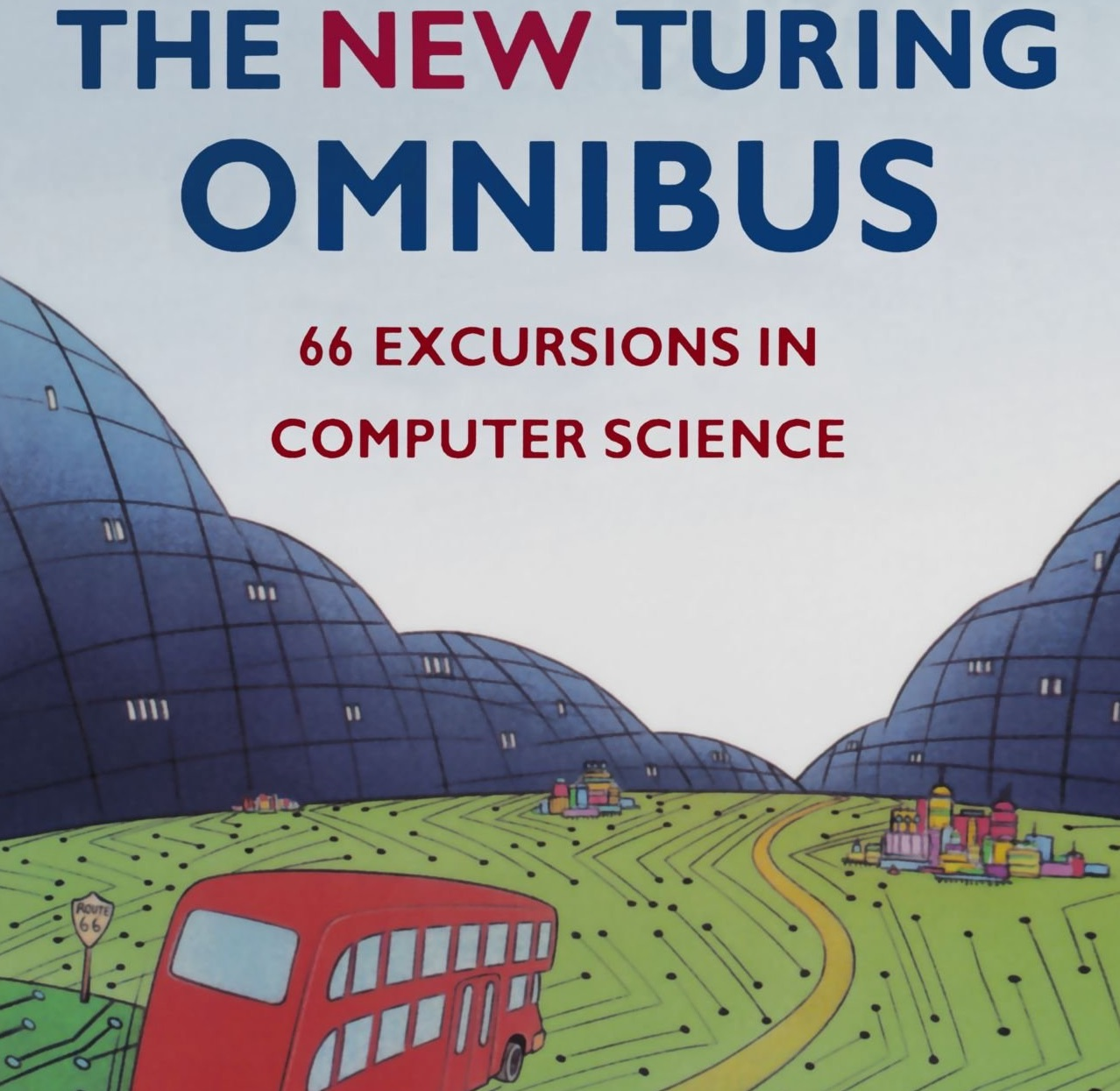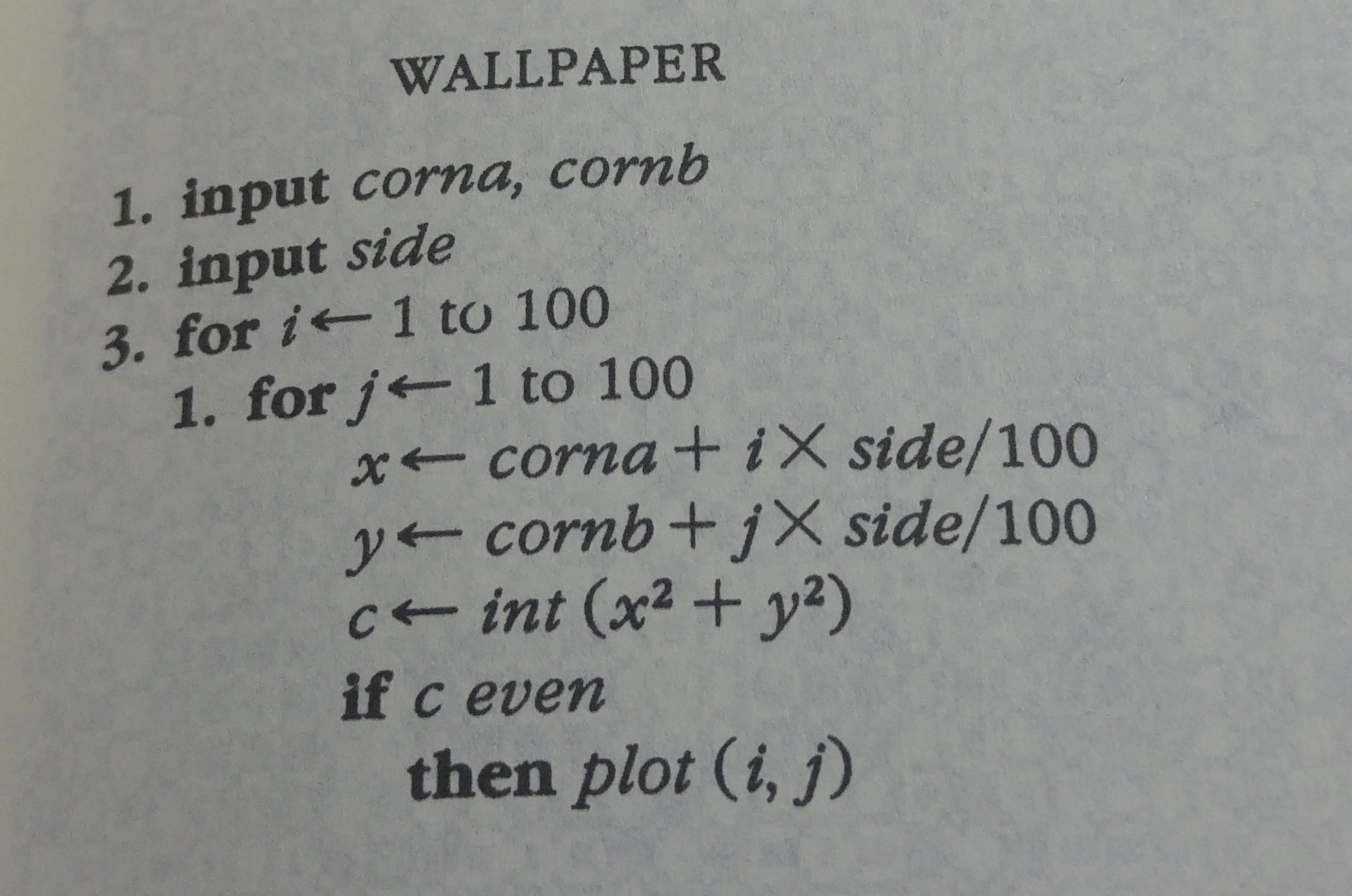I have started to read The New Turing Omnibus - a book that offers 66 concise, brilliantly written articles on the major points of interest in computer science theory, technology and applications.

From time to time, I will write a blog post presenting a chapter of this book.Today, I am glad to present an interactive version of Chapter 1 about algorithms in general.

## The algorithm

In order to explain what is an algorithm, the author presents a simple recipe for generating wallpapers.

Here is the recipe:Now, we are going to code this algorithm in `Clojurescript` and you will be able to play with it in your browser thanks to the interactive Klipse snippets.

## Preliminaries

First, we need a function that draws a color pixel on a canvas:

``````(defn draw-pixel! [canvas x y color]
(let [ctx (.getContext canvas "2d")
scale 2]
(set! (.-fillStyle ctx) color)
(.fillRect ctx (* scale x) (* scale y) scale scale)))
``````

Then, a function that erases a canvas i.e. color it in white:

``````(defn reset-canvas! [canvas]
(let [ctx (.getContext canvas "2d")]
(set! (.-fillStyle ctx) "white")
(.fillRect ctx 0 0 (.-width canvas) (.-height canvas))))
``````

## Black and White Wallpaper

The algorithm is controlled by the geometry of a square:

• its `x`-position named `a`
• its `y`-position named `b`
• the side of the square named `side`
``````

(defn draw-bw-wallpaper! [canvas a b side]
(let [points 200]
(dotimes [i points]
(dotimes [j points]
(let [x (+ a (* i (/ side points)))
y (+ b (* j (/ side points)))
c (int (+ (* x x) (* y y)))]
(when (even? c)
(draw-pixel! canvas i j "black")))))))

(draw-bw-wallpaper! canvas 5 5 9)
``````

The cool thing about this algorithm is that when we modify the side of the square, we get a completly different pattern:

``````
(draw-bw-wallpaper! canvas 5 5 100)
``````

Go ahead, play with the code…

The interactive code snippets are powered by the Klipse plugin.

## Three Colors

We can generate a 3-color wallpaper by calculating the remainder of `c` modulo 4 and chose a color accordingly:

``````

(defn draw-color-wallpaper! [canvas a b side]
(let [points 200]
(dotimes [i points]
(dotimes [j points]
(let [x (+ a (* i (/ side points)))
y (+ b (* j (/ side points)))
c (int (+ (* x x) (* y y)))
color  (case (mod c 4)
0 "red"
1 "green"
2 "blue"
"white")]
(draw-pixel! canvas i j color))))))

(draw-color-wallpaper! canvas 5 7 101)
``````

Again, when we modify the side of the square, we get a completly different pattern:

``````
(draw-color-wallpaper! canvas 5 7 57)
``````

## Grand Finale

Someone in reddit suggested to loop over the value of `side` in order to watch all the generated wallpapers like a movie.

Here is the result:

``````
(defonce interval (atom nil))
(defonce side (atom 0))

(def delta 0.5)
(defn step [canvas container]
(set! (.-innerHTML container) (str "side: " @side) )
(reset-canvas! canvas)
(draw-color-wallpaper! canvas 5 5 (swap! side + delta)))

(.clearInterval js/window @interval)
(reset! side 0)
(reset! interval (.setInterval js/window step  500 canvas js/klipse-container))
``````

Are you able to provide a simple explanation about this algorithm?

How is it able to generate so many different beautiful patterns?

Have you found a magnificient pattern? Please share its code…